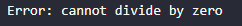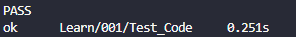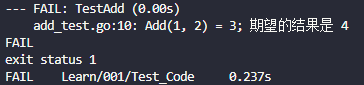#### 错误处理

`Go` 语言对错误处理有一个独特的方法。

``````package main

import (
"errors"
"fmt"
)

// 模拟一个可能出错的函数
func divide(dividend, divisor float64) (float64, error) {
if divisor == 0 {
return 0, errors.New("cannot divide by zero")
}
return dividend / divisor, nil
}

func main() {
result, err := divide(10, 0)
if err != nil {
fmt.Println("Error:", err)
return
}
fmt.Println("Result:", result)
}
``````#### 单元测试

`Go` 语言中，单元测试是通过内置的 `testing` 包和 `go test` 命令来实现的。🦌🔍

`Go` 中，一个单元通常是一个函数。

``````// add.go
package math

func Add(x, y int) int {
return x + y
}
``````

``````// add_test.go
package math

import "testing"

expected := 3
if result != expected {
t.Errorf("Add(1, 2) = %d; 期望的结果是 %d", result, expected)
}
}
``````

``````go test
````````````// add_test.go
package math

import "testing"

expected := 3
if result != expected {
t.Errorf("Add(1, 2) = %d; 期望的结果是 %d", result, expected)
}
}
``````#### 表格驱动测试

``````func TestAddTableDriven(t *testing.T) {
var tests = []struct {
x        int
y        int
expected int
}{
{1, 2, 3},
{1, 1, 2},
{2, 2, 4},
}

for _, tt := range tests {
testname := fmt.Sprintf("%d+%d", tt.x, tt.y)
t.Run(testname, func(t *testing.T) {
if ans != tt.expected {
t.Errorf("got %d, want %d", ans, tt.expected)
}
})
}
}
``````

`t.Run` 允许我们为每个测试用例运行一个子测试。微信支付宝